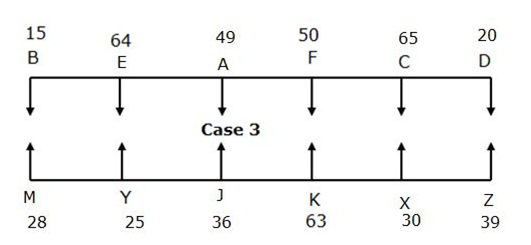# IBPS RRB Clerk Mains Reasoning Questions 2019 (Day-06)

Dear Aspirants, Our IBPS Guide team is providing new series of Reasoning Questions for IBPS RRB Clerk Mains 2019 so the aspirants can practice it on a daily basis. These questions are framed by our skilled experts after understanding your needs thoroughly. Aspirants can practice these new series questions daily to familiarize with the exact exam pattern and make your preparation effective.

Check here for IBPS RRB PO Mains Mock Test 2019

Check here for IBPS RRB Clerk Mains Mock Test 2019

Check here for IBPS PO Prelims Mock Test 2019

### Click Here to Subscribe Crack High Level Puzzles & Seating Arrangement Questions PDF 2019 Plan

[WpProQuiz 7114]

Directions (1-5):Study the given information carefully and answer the following questions.

Eight persons A, B, C, D, E, F, G and H are born in a month of July in different years. Their ages are calculated on the same month with respect to 2018.

If it mentions the person age is considered as last two digits of the person Birth year, then it will be at any sequence. For example, X age is considered as last two digit of Y birth year-1936, and then X age is either 36 or 63.

None of the person born before 1962.

F was born on 1968. The difference between the age of F and B was Five years. C’s age is two less than last two digit of Birth year of B. B’s age is one less than the sum of E’s and C’s age. A is three year elder than C. H’s age is last two digit of A’s birth year. G is four year younger than H. D is born Four year after G.

1) What is the age of D?

a) None of those given as options

b) 14

c) 10

d) 22

e) 38

2) Which of the following is the birth year of G?

a) 1994

b) 2014

c) 2000

d) None of those given as options

e) 2004

3) If I was born between A and C then which of the following can be the birth year of I?

a) 1980

b) None of those given as options

c) 1990

d) 1982

e) 1985

4) What will be the sum of ages of youngest and eldest person in the group?

a) 75 years

b) 65 years

c) 60 years

d) 49 years

e) None of those given as options

5) Who among them will be the fourth youngest person in the group?

a) E

b) B

c) None of those given as options

d) A

e) C

Directions (6-10): Study the given information carefully and answer the following questions.

Twelve persons are sitting in a two parallel row, Row 1 and Row 2. A, B, C, D, E, and F are sitting in row 1 and facing South direction. X, Y, Z, J, K, and M are sitting in row 1 and facing North direction. They have different ages viz, 15, 20, 25, 28, 30, 36, 39, 49, 50, 63, 64, and 65. The person whose age is even numbers are not sit adjacent to each other and the person whose age is the odd numbers are not sit adjacent to each other.

A is elder than J. K is 63 years old and sits third from the extreme end of the row. F’s age is twice that of Y and sits opposite to K. There is two person sits between F and B who is the youngest person among the group. Y sits opposite to the one whose age is 64 years. C’s age is multiple of 5 and D sits immediate left of C. D is not 64 years old. X is 20 years younger than F and sits opposite to C. The age of A and J is a square number below 50. M sits somewhere to the left of Y. A sits second to the left of the one who is opposite to M. The number of persons sits right of E is one less than that of the number of persons sits left of the one whose age is 36. The one whose age is 65 sits immediate right of the one whose age is 20 years old. Y is not the eldest person. Z is elder than J. Y is younger than M.

6) What will be the age of Z?

a) 25

b) 49

c) 36

d) 39

e) None of those given as options

7) How many people younger than Y?

a) One

b) Two

c) Three

d) More than three

e) Y is the youngest person

8) Who sits second to the left of the one who sits opposite to J?

a) The one who is the eldest person

b) The one who is 49 years old

c) The one who is youngest person

d) None of those given as option

e) D

9) Four of the five among the following are similar in such a way to form a group, which one of the following doesn’t belong to the group?

a) 15

b) 28

c) 39

d) 50

e) 20

10) What is the sum of ages of M, E and Z?

a) 121

b) 131

c) 148

d) 139

e) None of those given as options

Directions (1-5):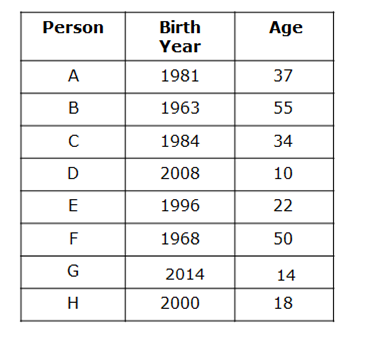Note:None of the person born before 1962.

• If it mentions the person age is considered as last two digits of the person Birth year, then it will be at any sequence. For example, X age is considered as last two digit of Y birth year-1936, and then X age is either 36 or 63.
• F was born on 1968.
• The difference between the age of F and B was Five years. So B can be born in either 1973 or 1963. So we have two possible cases.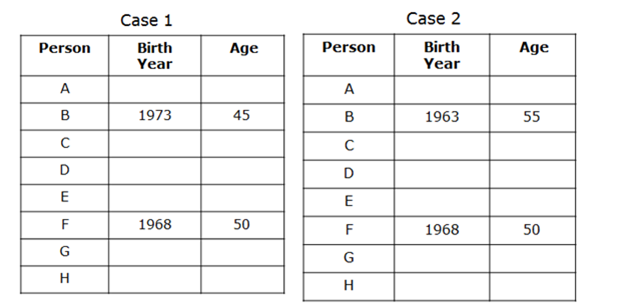• C’s age is two less than last two digit of Birth year of B. Here mention that C’s age is equal to the last two digits of B’s birth year. In both the cases if we take last two digit of B’s birth year in given sequence,then we get C born before 1962. So if we take reverse of last two digits of B’s birth year then it satisfies the given conditions. So C was born on 1983 in Case1 and 1984 in case 2.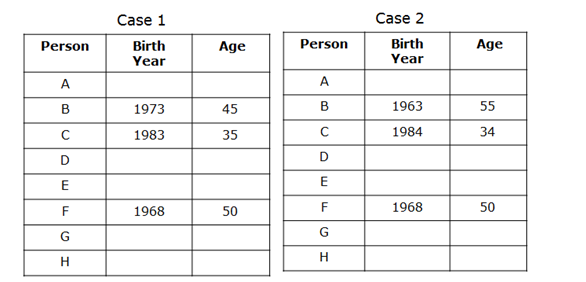• B’s age is one less than the sum of E’s and C’s age. We know that ages of B and C from that we can conclude the age of E as 11 and 22 in case 1 and case 2 respectively.
• A is three year elder than C. Hence the age of A is 38 and 37 in case 1 and case 2 respectively.
• H’s age is last two digit of A’s birth year. It may be 80 or 8 years in case 1 and 18 or 81 in Case 2. But from the note given above H’s age is fixing as 8 and 18 for case 1 and case 2.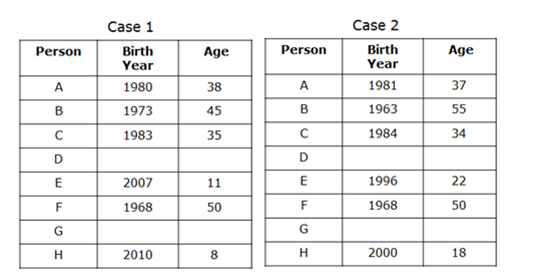• G is four year younger than H. From this G is 4 year old in case 1 and 14 years old Case 2.
• D is born Four year after G. Hence D is born on 2008 in case 2. But in Case 1 we cannot conclude the birth year of D. Hence Case 1 is eliminated.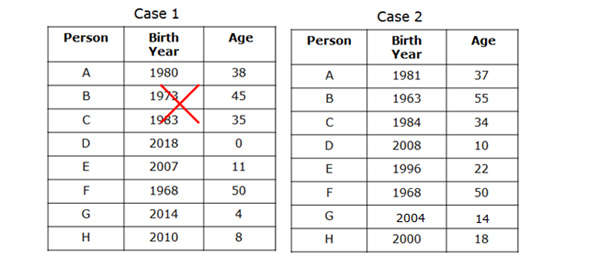Directions (6-10):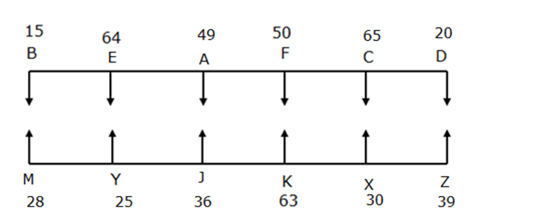• K is 63 years old and sits third from the extreme end of the row.
• F’s age is twice that of Y and sits opposite to K.
• There are two person sits between F and B who is the youngest person among the group.
• Y sits opposite to the one whose age is 64 years.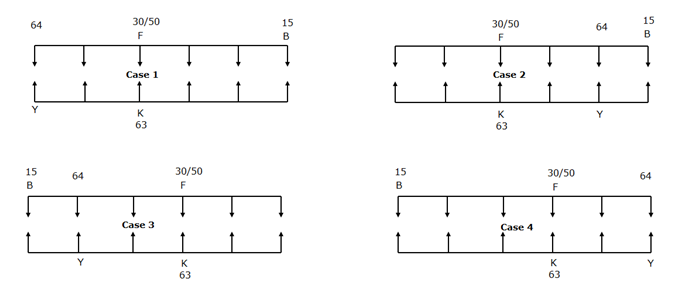• C’s age is multiple of 5 and D sits immediate left of C.
• D is not 64 years old. From this statement case 2 and Case 4 is eliminated.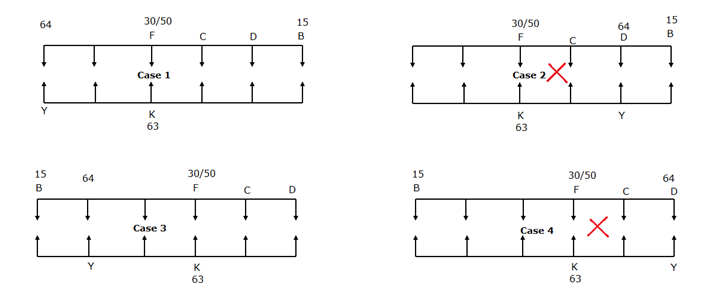• M sits somewhere to the left of Y. From this statement case 1 is eliminated.
• X is 20 year younger than F and sits opposite to C. Form that X age is 30years and F age should be 50.
• A sits second to the left of the one who is opposite to M.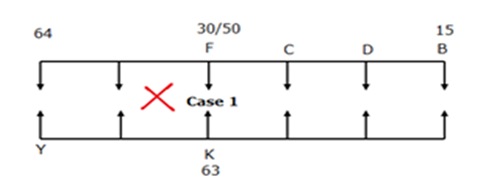Incase1, M sits somewhere to the left of Y, A sits second to the left of the one who is opposite to M, these conditions are not satisfied so rejected.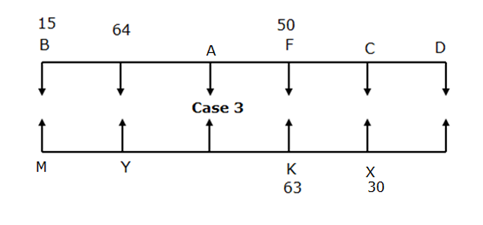• The number of person sits right of E is one less than that of number of person sits left of the one whose age is 36.E should be sits second from the right end in row 1.So we can fix the position of the one whose age is 36 at immediate right of Y.
• The age of A and J is a square number below 50.So it should be either 25 or 36 or 49.
• A is elder than J.
• From the above two statement J should be 36 years old and A should be 49 years old.
• The one whose age is 65 sits immediate right of the one whose age is 20 years old. Y is not the eldest person. From this statement D is 20 years old and C is 65 years old.
• Z elder than J. So Z Should be 39 years old
• Y is younger than M. so Y should be 25 years old and m should be 28 years old.